Circumference of circle

Chapter 9 Class 7 Perimeter and Area
Concept wise

Let us take a circle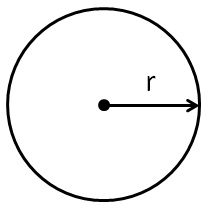Distance around the circle is called circumference of circle.

So, Perimeter of circle is actually circumference of circle.

For a circle with radius r,

Circumference = 2𝜋r

Here,

and

𝜋 = pi

It’s value is

𝜋 = 3.14159265359……

We write it as

𝜋 = 3.14 or 𝜋 = 22/7

Let’s take some examples

### Find circumference of circle with radius 3.5 cm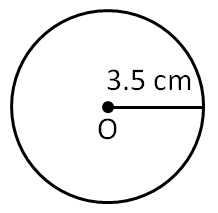= 3.5 cm

Circumference = 2𝜋r

= 2 × 22/7 × 3.5

= 2 × 22/7 × 35/10

= 2 × 22/1 × 5/10

= 22 × 5/5

= 22 × 1 = 22 cm

#### Find circumference of circle with radius 7.0 cm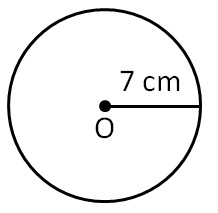= 7 cm

Circumference = 2𝜋r

= 2 × 22/7 × 7

= 2 × 22/1 × 1

= 44 cm

#### Find circumference of circle with diameter 21 cm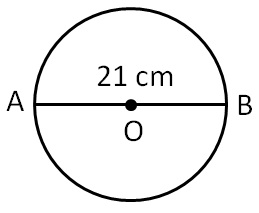Diameter = 21 cm

= 21/2

Circumference = 2𝜋r

= 2 × 22/7 × 21/2

= 22/7 × 21/1

= 22 × 3

= 66 cm

#### Find circumference of circle with diameter 42 cm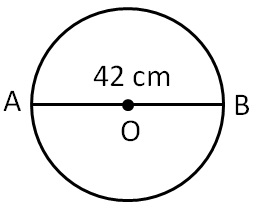Diameter = 42 cm

= 42/2

= 21 cm

Circumference = 2𝜋r

= 2 × 22/7 × 21

= 2 × 22/1 × 3

= 44 × 3

= 132 cm

#### Find circumference of circle with radius 5 cm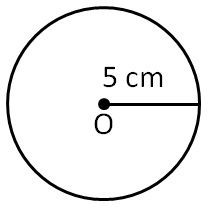= 5 cm

Circumference = 2𝜋r

= 2 × 3.14 × 5

= 3.14 × 5 × 2

= 3.14 × 10

= 31.4 cm

#### Find circumference of circle with diameter 30 cm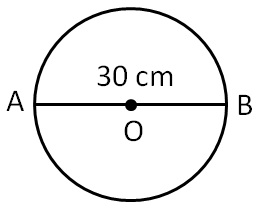Diameter = 30 cm

= 30/2

= 15 cm

Circumference = 2𝜋r

= 2 × 3.14 × 15

= 3.14 × 2 × 15

= 314/100 × 30

= 314/10 × 3

= 942/10

= 94.2 cm

Learn in your speed, with individual attention - Teachoo Maths 1-on-1 Class